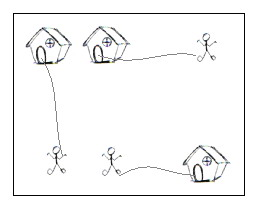# POJ-2195 Going Home 【费用流】

Description

On a grid map there are n little men and n houses. In each unit time, every little man can move one unit step, either horizontally, or vertically, to an adjacent point. For each little man, you need to pay a \$1 travel fee for every step he moves, until he enters a house. The task is complicated with the restriction that each house can accommodate only one little man.

Your task is to compute the minimum amount of money you need to pay in order to send these n little men into those n different houses. The input is a map of the scenario, a '.' means an empty space, an 'H' represents a house on that point, and am 'm' indicates there is a little man on that point.You can think of each point on the grid map as a quite large square, so it can hold n little men at the same time; also, it is okay if a little man steps on a grid with a house without entering that house.

Input

There are one or more test cases in the input. Each case starts with a line giving two integers N and M, where N is the number of rows of the map, and M is the number of columns. The rest of the input will be N lines describing the map. You may assume both N and M are between 2 and 100, inclusive. There will be the same number of 'H's and 'm's on the map; and there will be at most 100 houses. Input will terminate with 0 0 for N and M.

Output

For each test case, output one line with the single integer, which is the minimum amount, in dollars, you need to pay.

Sample Input

2 2
.m
H.
5 5
HH..m
.....
.....
.....
mm..H
7 8
...H....
...H....
...H....
mmmHmmmm
...H....
...H....
...H....
0 0

Sample Output

2
10
28

题解：最小费用最大流，相邻点连边容量inf，费用1，设源点到有m的点(1,0), 设H的点到汇点(1,0)。代码：
  1 #include<iostream>
2 #include<algorithm>
3 #include<cstring>
4 #include<queue>
5 #include<stack>
6 #include<cstdio>
7 using namespace std;
8 #define INF 0x3f3f3f3f
9 #define M(a, b) memset(a, b, sizeof(a))
10 const int N = 1e4 + 5;
11 const int dx[] = {-1, 1, 0, 0};
12 const int dy[] = {0, 0, -1, 1};
13 int n, m;
14 struct Edge {
15     int from, to, cap, flow, cost;
16 };
17
18 struct MCMF {
19     int n, m;
20     vector<Edge> edges;
21     vector<int> G[N];
22     int d[N], inq[N], p[N], a[N];
23
24     void init(int n) {
25         this->n = n;
26         for (int i = 0; i <= n; ++i) G[i].clear();
27         edges.clear();
28     }
29
30     void AddEdge(int from, int to, int cap, int cost) {
31         edges.push_back((Edge){from, to, cap, 0, cost});
32         edges.push_back((Edge){to, from, 0, 0, -cost});
33         m = edges.size();
34         G[from].push_back(m-2); G[to].push_back(m-1);
35     }
36
37     bool spfa(int s, int t, int &flow, int &cost) {
38         M(inq, 0); M(d, INF);
39         d[s] = 0; inq[s] = 1; p[s] = 0; a[s] = INF;
40         queue<int> q;
41         q.push(s);
42         while (!q.empty()) {
43             int x = q.front(); q.pop();
44             inq[x] = 0;
45             for (int i = 0; i < G[x].size(); ++i) {
46                 Edge &e = edges[G[x][i]];
47                 if (d[e.to] > d[x] + e.cost && e.cap > e.flow) {
48                     d[e.to] = d[x] + e.cost;
49                     p[e.to] = G[x][i];
50                     a[e.to] = min(a[x], e.cap-e.flow);
51                     if (inq[e.to]) continue;
52                     q.push(e.to); inq[e.to] = 1;
53                 }
54             }
55         }
56         if (d[t] == INF) return false;
57         flow += a[t];
58         cost += d[t] * a[t];
59         int u = t;
60         while (u != s) {
61             edges[p[u]].flow += a[t];
62             edges[p[u]^1].flow -= a[t];
63             u = edges[p[u]].from;
64         }
65         return true;
66     }
67
68     int Mincost(int s, int t) {
69         int flow = 0, cost = 0;
70         while (spfa(s, t, flow, cost));
71         return cost;
72     }
73
74 }solver;
75 char str;
76
77 bool check(int x, int y) {
78     if (x>0 && x<=n && y>0 && y<=m) return 1;
79     return 0;
80 }
81
82 int Hash(int i, int j) {return (i-1)*m+j;}
83
84 int main() {
85     while (scanf("%d%d", &n, &m), n&&m) {
86         solver.init(n*m+1);
87         for (int i = 1; i <= n; ++i) {
88             scanf("%s", str[i]+1);
89             for (int j = 1; j <= m; ++j) {
90                 if (str[i][j]=='H') solver.AddEdge(Hash(i, j), n*m+1, 1, 0);
91                 if (str[i][j]=='m') solver.AddEdge(0, Hash(i, j), 1, 0);
92                 for (int k = 0; k < 4; ++k) {
93                     int nx = i+dx[k], ny = j+dy[k];
94                     if (check(nx, ny)) solver.AddEdge(Hash(i, j), Hash(nx, ny), INF, 1);
95                 }
96             }
97         }
98         printf("%d\n", solver.Mincost(0, n*m+1));
99     }
100
101     return 0;
102 }

posted @ 2017-05-02 20:37  Robin!  阅读(119)  评论(0编辑  收藏